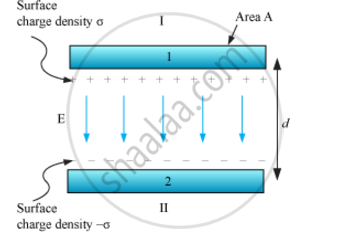Share

# Derive the Expression for the Capacitance of a Parallel Plate Capacitor Having Plate Area a and Plate Separation D - Physics

ConceptCapacitance of a Parallel Plate Capacitor with and Without Dielectric Medium Between the Plates

#### Question

Derive the expression for the capacitance of a parallel plate capacitor having plate area A and plate separation d.

#### Solution

A parallel plate capacitor consists of two large plane parallel conducting plates separated by a small distance.Let A be the area of each plate and d be the separation between them. The two plates have charges Q and −Q. Plate 1 has surface charge density, σ = Q/A,
And plate 2 has a surface charge density −σ.

Electric field in different regions:

Outer region I:

E= sigma/(2epsilon_0)-sigma/(2epsilon_0)=0

Outer region II:

E=sigma/(2epsilon_0)-sigma/(2epsilon_0)=0

In the inner region between plates 1 and 2, the electric fields due to the two charged plates add up.

This gives:

E=sigma/(2epsilon_0)+sigma/(2epsilon_0)=sigma/epsilon_0=Q/epsilon_0=Q/(epsilon_0A)

The direction of electric field is from positive to the negative plate. For uniform electric field, potential difference is simply the electric field times the distance between the plates.

V=E""d=1/epsilon_0(Qd)/A

Capacitance (C) of the parallel plate capacitor, C=Q/V=(epsilon_0A)/d

Is there an error in this question or solution?

#### Video TutorialsVIEW ALL 

Solution Derive the Expression for the Capacitance of a Parallel Plate Capacitor Having Plate Area a and Plate Separation D Concept: Capacitance of a Parallel Plate Capacitor with and Without Dielectric Medium Between the Plates.
S